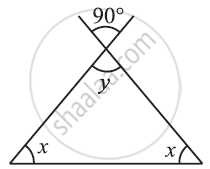# Find the Value of the Unknowns X And Y In the Following Diagram: - 5 - Mathematics

#### Question

Find the value of the unknowns x and y in the following diagram:#### Solution

y = 90° (Vertically opposite angles)

y = 180° (Angle sum property)

2x + y = 180°

2x + 90° = 180°

2x = 180° − 90° = 90°

x = 90^@/2 = 45^@

Is there an error in this question or solution?

#### APPEARS IN

NCERT Solution for Mathematics for Class 7 (2018 (Latest))
Chapter 6: The Triangle and its Properties
Exercise 6.3 | Q 2.5 | Page 121

#### Video TutorialsVIEW ALL 

Find the Value of the Unknowns X And Y In the Following Diagram: - 5 Concept: Angle Sum Property of a Triangle.